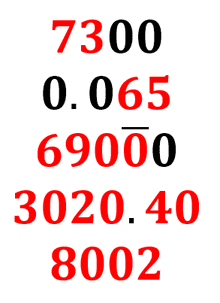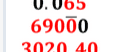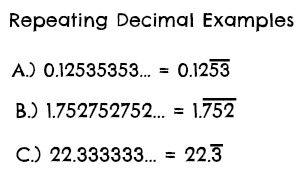# A bit confused about significant figures

• Featured
maxelcat
Homework Statement:
How many sig fig does the a number like 10 have?
Relevant Equations:
20 has 1 sig fig
Hi. I understand that the number 20, written like this, has 1 significant figure

Suppose I took a pile of measures of length: 18, 19, 20, 21, 22, 23, 24, 25 giving a mean of 172/8 = 21.5 on my calculator

If I have to give the mean to the lowest number of significant figures then that would give an answer of 20 - which feels wrong.

I think I should work to 2 sig fig so 22 - which makes sense. So I guess my question is how how many sig fig does 20 have? Can it depend on the context?

Homework Helper
Gold Member
2022 Award
So I guess my question is how how many sig fig does 20 have? Can it depend on the context?
Yes, it can be ambiguous. If you really want to be precise, you need to add a note or comment.

For example, if you have data to three significant figures you may write ##20.0##, rather than simply ##20##.

For ##20## itself I think you would have to write ##20## (two significant figures) to be clear.

Homework Helper
Gold Member
Strictly speaking, if you wanted to write the number to one significant figure, you would use ##2\times 10^1##. To two significant figures, it would be ##2.0\times 10^1##, to three ##2.00\times 10^1## and so on. That makes the intended number of significant figures clear, but the result is not aesthetically pleasing.

•FactChecker, phinds and berkeman
archaic
Strictly speaking, if you wanted to write the number to one significant figure, you would use ##2\times 10^1##. To two significant figures, it would be ##2.0\times 10^1##, to three ##2.00\times 10^1## and so on. That makes the intended number of significant figures clear, but the result is not aesthetically pleasing.
I think that ##20.## is also used for two significant figures.

•Dale
Gold Member
I've seen suggestions of including the period to make it clear that it has two significant figures (##20.##).

Personally, I would assume ##20## has always two significant figures. If there was only one, the appropriate way of writing it would be ##2 \times 10^1## or ##2E01##. If there is a unit, you can also change the prefix. (Ex.: use ##2\ cm## instead of ##20\ mm##)

As for your example for calculating the average, using numbers between 18 and 25 clearly indicates that they have 2 sig fig, so 20 must have it too.

•FactChecker and berkeman
Homework Helper
Gold Member
From https://en.wikipedia.org/wiki/Significant_figures
Wikipedia said:Digits in red are significant figures; those in black are not

•berkeman
Homework Helper
Gold Member
The inset from Wikipedia is not very informative as shown because it leads one to believe that the sig figs can be determined simply by looking at the number. Is the number quoted by OP 20 or 20? That's the ambiguity mentioned by @PeroK.

Wikipedia correctly points out "Zeros to the right of the significant figures are significant if and only if they are justified by the precision of their derivation." Simply writing down a 2 followed by a 0 says nothing about the precision of the derivation and is inherently ambiguous.

Mentor
I like the Wikipedia article, but I was also a bit confused when I saw the overbar notation being used in this context. I guess it must be a standard notation for significant digits and marking the zeros?But when I first saw it, my mind immediately went to "repeating digits" notation, which sent me way off into the weeds...https://study.com/cimages/multimages/16/repeating_decimals_examples_1.jpgHomework Helper
2022 Award
If it really matters I think it prudent to write the number correctly using scientific notation. Every time I see this or similar questions, I get at least one of the example standard definitions wrong ! Occasionally this could be problematic...

•Dale and berkeman
Gold Member
•DifferentialGalois and Dale
maxelcat
Dear All - thanks for the interesting comments above. The situation has arisen as I was going over a physics question with a 13 year old - though tbh this has been nagging away at me for some time. Searching didn't help... I think (actually I know!) the use of standard form might be too much for a lot of school kids these days... but those who can handle it could deal with it. Very helpful - thanks again!

Gold Member
So - long story short - "significant figures" is the length of the mantissa. In practice, how is it different from "precision" ?

In practice, how is it different from "precision" ?

All there is to it is that the significant figures printed reflect the precision of the measurement. Many authors employ different conventions, but a common one is that the final significant digit is the uncertain/estimated digit w.r.t. the precision of the measuring apparatus e.g. ##31.6 \text{cm} \pm 0.5 \text{cm}##.

•hmmm27
Homework Helper
So - long story short - "significant figures" is the length of the mantissa. In practice, how is it different from "precision" ?
Yes. It matches the length of the base 10 mantissa for a floating point value. This is, in turn, a crude way to express relative precision.

Crudely:

1 significant digit = precise to 10%.
2 significant digits = precise to 1%.
3 significant digits = precise to 0.1%
and so on.

One reason that this measure is crude is that "1.1" has 2 significant figures. The true value could be anywhere between 1.15 (+5%) and 1.05 (-5%). Meanwhile "9.8" also has 2 significant figures. The true value could be anywhere between 9.85 (+0.5%) and 0.975 (-0.5%).

So does 2 significant figures mean 5% relative precision? Or 0.5% relative precision? My answer is to say 1% and call it "crude".

•Dale and hutchphd
Gold Member
My answer is to say 1% and call it "crude".
The same problem as "orders of magnitude".

Mentor
If it really matters I think it prudent to write the number correctly using scientific notation.
If it really matters give actual uncertainties (##\pm x##). Significant figures can never give a good uncertainty estimate, they can only stop you from adding a silly number of digits that have no relevance to the answer.

•DifferentialGalois, Dale, dlgoff and 1 other person
Gold Member
If it really matters give actual uncertainties (##\pm x##). Significant figures can never give a good uncertainty estimate, they can only stop you from adding a silly number of digits that have no relevance to the answer.

Agreed. Significant figures aren't more than an upper bound on the uncertainty. Further, the uncertainty in a mean value can often be SMALLER than the uncertainty in individual values that went into it. The conventions with significant figures ignore this possibility.

In this case, a better way to denote the mean would be 21.5 ##\pm 0.8##.

The uncertainty of 0.8 is estimated as the standard error of the mean.

https://en.wikipedia.org/wiki/Standard_error

I've never regarded the rules of significant digits as either a rigorous way to determine uncertainties nor a good way to communicate them. The rules of significant digits also ignore how errors propagate differently depending on how the number is used in an equation. If a number is squared a 1% uncertainty becomes a 2% uncertainty. If the square root of a number is taken, a 1% uncertainty becomes a 0.5% uncertainty.

I don't usually teach the conventions of significant digits. My experience is that these conventions tend to make students overconfident in the accuracy of their results. For class type problem solving, I usually just ask students to give 2 or 3 digits past the decimal point in their final answers and use 3-4 digits past the decimal point for intermediate results. For lab computations with measured values, I instruct students to use a sensible number of significant digits based on the accuracy of the equipment, and I present a simple yet sensible method for estimating uncertainties based on the given experiment.

•etotheipi and PeroK
Homework Helper
2022 Award
For class type problem solving, I usually just ask students to give 2 or 3 digits past the decimal point in their final answers and use 3-4 digits past the decimal point for intermediate results.
Probably a pretty good way to go. The alternative is to try to keep your head from exploding when the student dutifully records 7 significant digits on an answer that is off by six orders of magnitude...

Mentor
Homework Statement:: How many sig fig does the a number like 10 have?
Relevant Equations:: 20 has 1 sig fig

Suppose I took a pile of measures of length: 18, 19, 20, 21, 22, 23, 24, 25 giving a mean of 172/8 = 21.5 on my calculator
Significant figures is intended to be a "quick and dirty" way to approximate uncertainty. Assuming that you did everything well and instrument gradations is your source of uncertainty, when you say you measured 18, what you probably mean that the measurand is somewhere in the range 17.5 to 18.5. Usually a uniform distribution is a reasonable assumption, leading to a standard uncertainty of 0.29. So written long ways it would be 18.00 ± 0.29 or, more compactly 18.00(29). Basically, significant figures just chops off any digits with uncertainty.

Now, the propagation of errors says that when you add numbers the uncertainty adds in quadrature (square root of the sum of squares) so the sum of your data is 172 and the standard uncertainty is ##\sqrt{8*0.29^2}=0.82##. Then 8 does not have any uncertainty, so the standard uncertainty of the mean is 0.10 and we would write it 21.5 ± 0.10 or more compactly 21.50(10).

This gives you more information about the actual uncertainty in the average than you get by just writing 21. But as you can see, if you chop off any digits with uncertainty then you do indeed get 21.

Last edited: